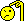View New Posts
1. ##March 22, 2022 = 13 and 666

03/22/2022

3+2+2+2+2+2= 13

322 = Skull & Bones/Brotherhood of Death

March is 3

22 is 22...

3 (March) *22 (Day) =66 + the year...

2022 = 6 (2+2+2=6) - March 22, 2022 = 666.Reply With Quote

2. ##Originally Posted by SnowballMarch is 3

22 is 22...

3 (March) *22 (Day) =66 + the year...

2022 = 6 (2+2+2=6) - March 22, 2022 = 666.Wouldn’t it be easier (and more believable) if you just wait until June 6th?
June is 6

The 6th is 6

2022 = 6 (2+2+2=6) - June 6th, 2022 = 666.Reply With Quote

3. ##Halloween always scares meReply With Quote

4. ##(3+2+2) = 7 and (2+0+2+2) = 6 so 7 and 6. Take 76ers -7 tonight.
Nomination(s):Reply With Quote 2

5. ##And the moon will be a waning gibbous.

Waning is 6 letters.
G is the 6th letter of alphabet
There are 6 letters after the g in gibbous. 666Reply With Quote

6. ##Originally Posted by Foxx(3+2+2) = 7 and (2+0+2+2) = 6 so 7 and 6. Take 76ers -7 tonight.
and they won by 15.Reply With Quote

7. ##Originally Posted by pablo222And the moon will be a waning gibbous.

Waning is 6 letters.
G is the 6th letter of alphabet
There are 6 letters after the g in gibbous. 666
savant stuffReply With Quote

8. ##Originally Posted by Snowball22 is 22...
This is deep stuff.Reply With Quote